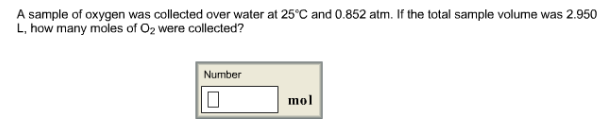# Problem: A sample of oxygen was collected over water at 25°C and 0.852 atm. If the total sample volume was 2.950 L, how many moles of O2 were collected?

###### FREE Expert Solution
95% (314 ratings)###### Problem Details

A sample of oxygen was collected over water at 25°C and 0.852 atm. If the total sample volume was 2.950 L, how many moles of O2 were collected?